Question

# A three-phase system is shown in the figure below, with line-to-line voltage of 220 V rms,...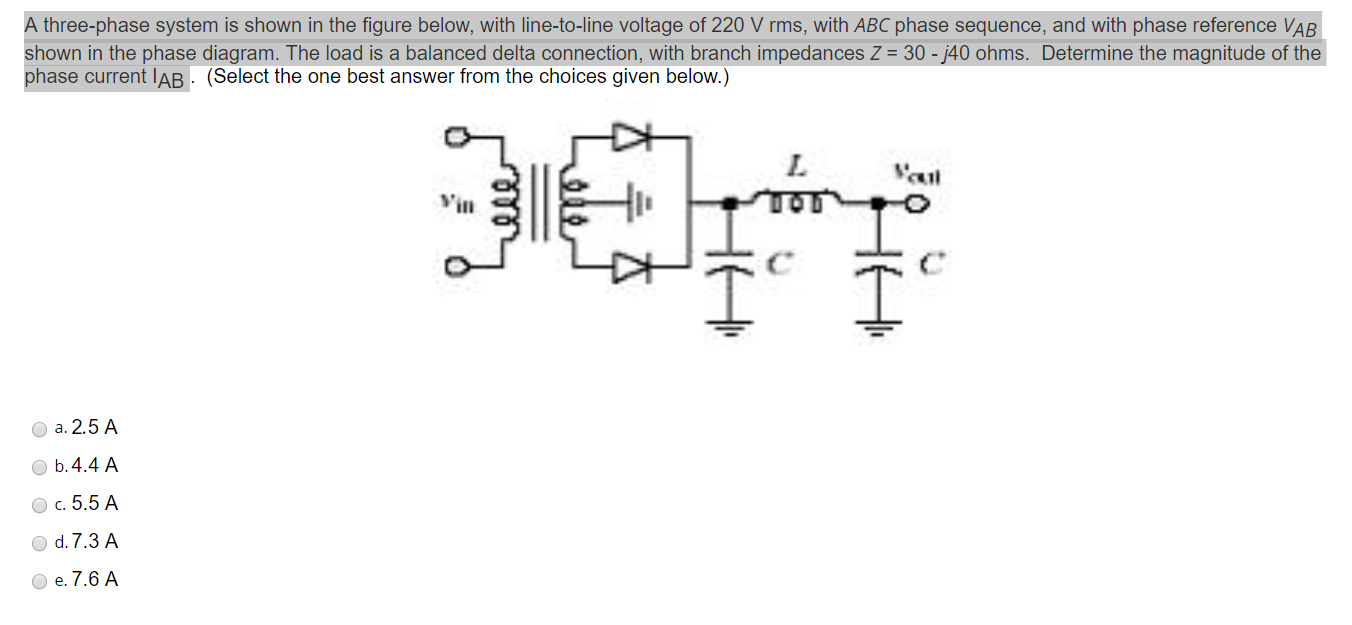A three-phase system is shown in the figure below, with line-to-line voltage of 220 V rms, with ABC phase sequence, and with phase reference VAB shown in the phase diagram. The load is a balanced delta connection, with branch impedances Z = 30 - 40 ohms. Determine the magnitude of the phase current IAB (Select the one best answer from the choices given below.) L ail HA Oo oo a. 2.5 A b.4.4 A c.5.5 A O d.7.3 A e. 7.6 A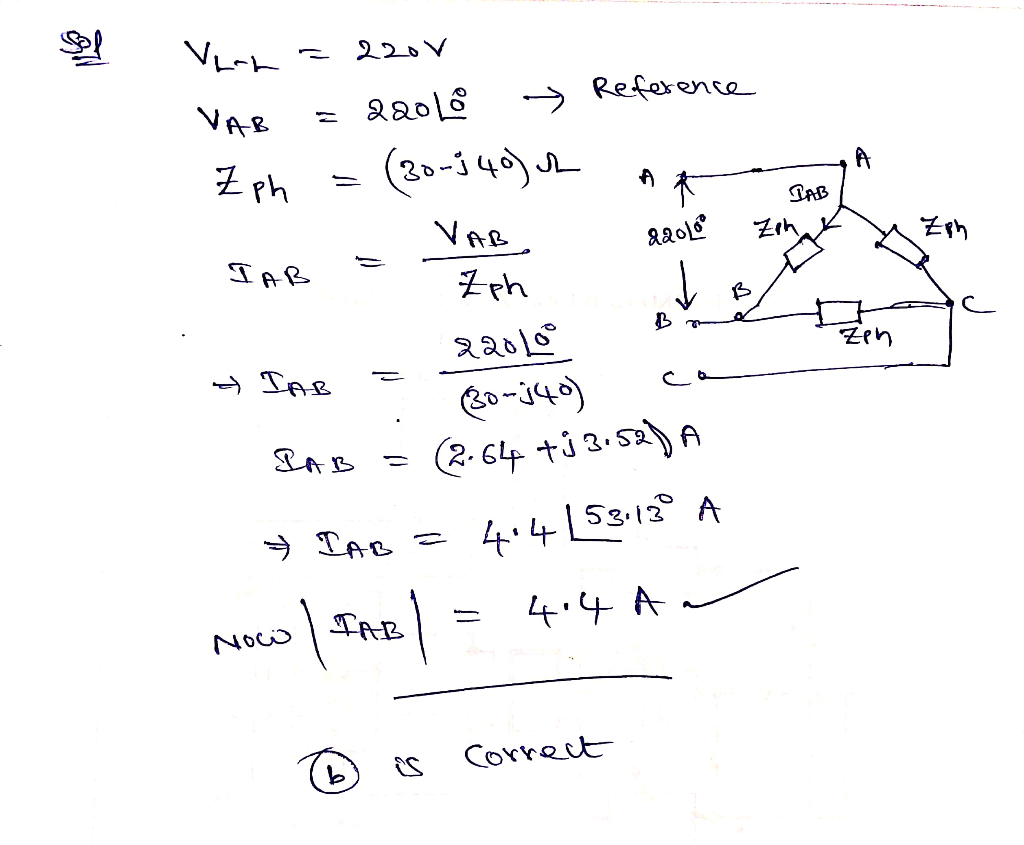#### Earn Coins

Coins can be redeemed for fabulous gifts.

Similar Homework Help Questions
• ### QUESTION 4: A 400 V three-phase, 50 Hz system, ABC sequence supplies the following loads: A...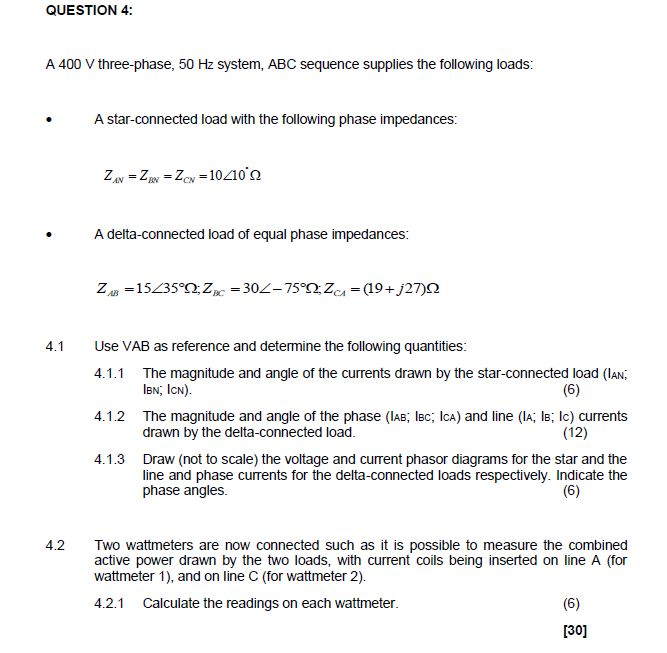QUESTION 4: A 400 V three-phase, 50 Hz system, ABC sequence supplies the following loads: A star-connected load with the following phase impedances: ZA ZZ10410' BN A delta-connected load of equal phase impedances: ZB15435Z=30Z-75Zc=(19+j27 )n 4.1 Use VAB as reference and detemine the following quantities: The magnitude and angle of the currents drawn by the star-connected load (lAN lEN, ICN) 4.1.1 (6) 4.1.2 The magnitude and angle of the phase (IAB; lBc; IcA) and line (IA; lB; Ic) currents drawn...

• ### a) Find the magnitude of the line current IaA (A rms) b) Find the phase of...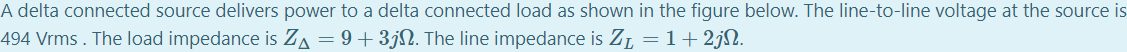a) Find the magnitude of the line current IaA (A rms) b) Find the phase of the line current IaA. (Phase deg) c) Find the magnitude of VAB the line-to-line load voltage (V rms) d) Find the phase of VAB (deg) e) Find the magnitude of the load phase current IAB (A rms) f) Find the phase of IAB (deg) g) Find the total power delivered to the load (kW) h) Find the power lost in the line. (kW) A...

• ### In a balanced three-phase Y-A system, the source has an ABC phase sequence and Van=114/_43° V...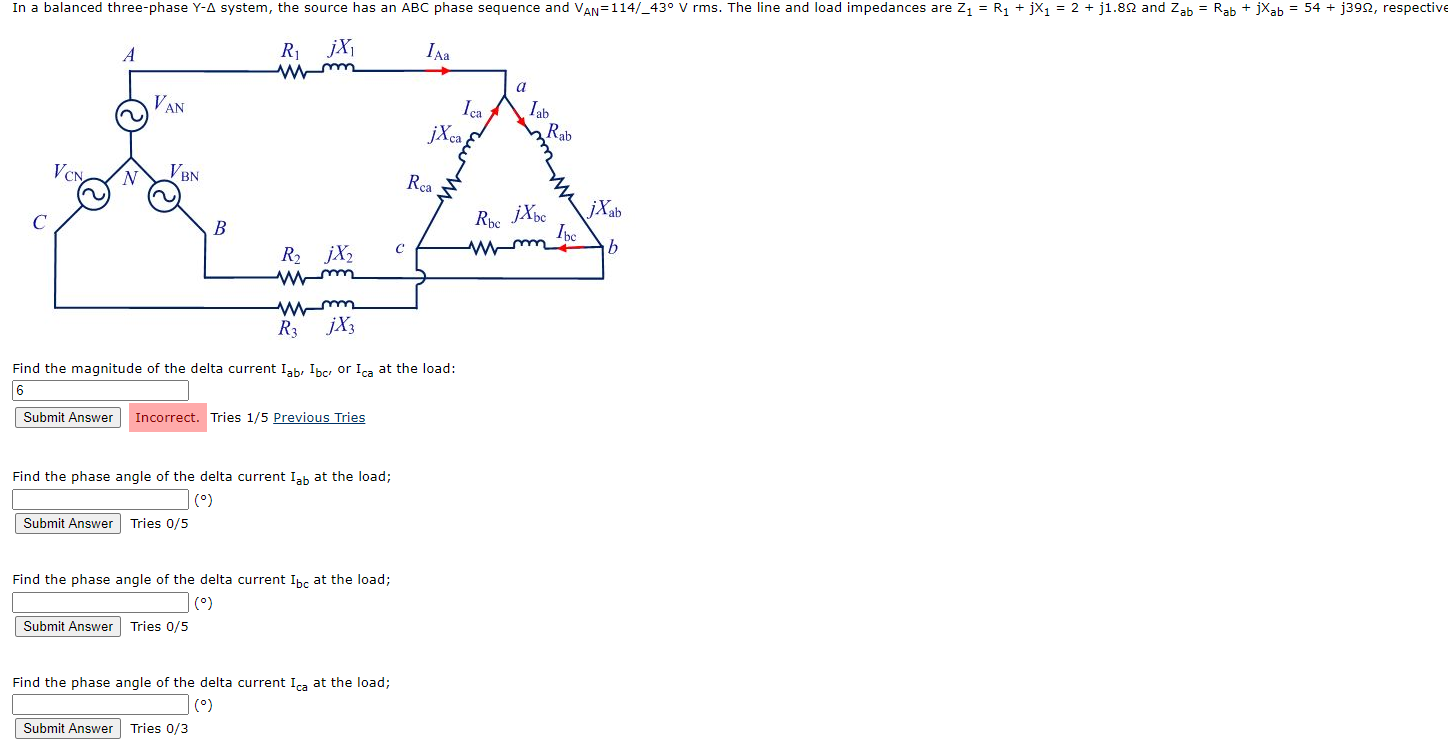In a balanced three-phase Y-A system, the source has an ABC phase sequence and Van=114/_43° V rms. The line and load impedances are Z1 = R1 + jX1 = 2 + j1.82 and Zab = Rab + jXab = 54 + j3922, respective А RijX w IAa a VAN n Lab Ica јХca, Rab VON N VBN Rca jXab C B Roc jXbo с b RjX2 Wm Wm R3 jX3 Find the magnitude of the delta current Iab, Ibc, or...

• ### ELE2601/101/3/2019 QUESTION 4 A 400 V three-phase, 50 Hz system, ABC sequence supplies the following loads: A star-connected load with the following phase impedances: A delta-connected load of eq...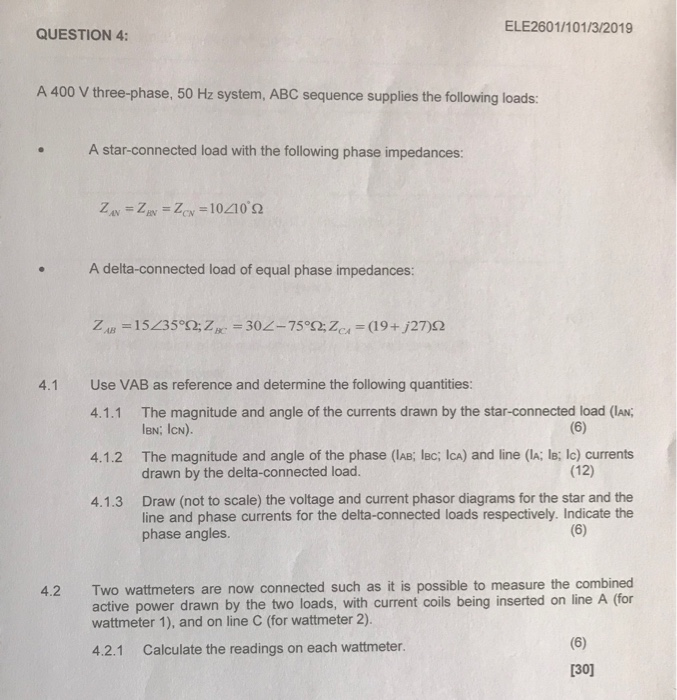ELE2601/101/3/2019 QUESTION 4 A 400 V three-phase, 50 Hz system, ABC sequence supplies the following loads: A star-connected load with the following phase impedances: A delta-connected load of equal phase impedances: Use VAB as reference and determine the following quantities: 4.1 The magnitude and angle of the currents drawn by the star-connected load (IAN; lBN; ICN). 4.1.1 The magnitude and angle of the phase (IAB; lBc; ICa) and line (lA; ls; Ic) currents drawn by the delta-connected load. 4.1.2 (12)...

• ### connected 3-phase load consisting of three identical impedances Z= 48ے36.870 W. 3-Phase System Using MATLAB/Simulink Analysis of Y-Y connected balanced 3-phase circuit using Matlab A 2400-V...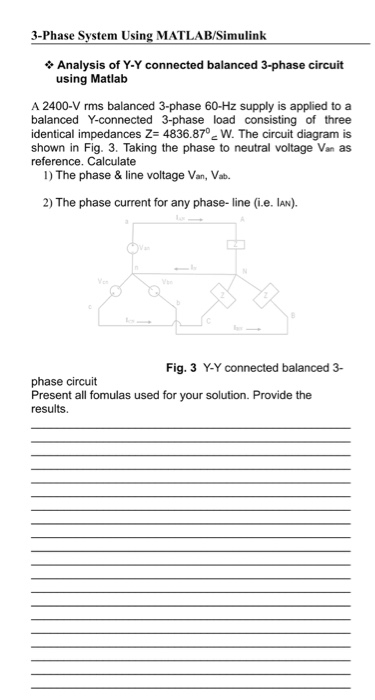connected 3-phase load consisting of three identical impedances Z= 48ے36.870 W. 3-Phase System Using MATLAB/Simulink Analysis of Y-Y connected balanced 3-phase circuit using Matlab A 2400-V rms balanced 3-phase 60-Hz supply is applied to a balanced Y-connected 3-phase load consisting of three identical impedances Z- 4836.870 W. The circuit diagram is shown in Fig. 3. Taking the phase to neutral voltage Van as reference. Calculate 1) The phase &line voltage Van, Vab 2) The phase current for any phase- line...

• ### A delta-wye connected transformer is shown in Figure below. This is a 220 kV to 450...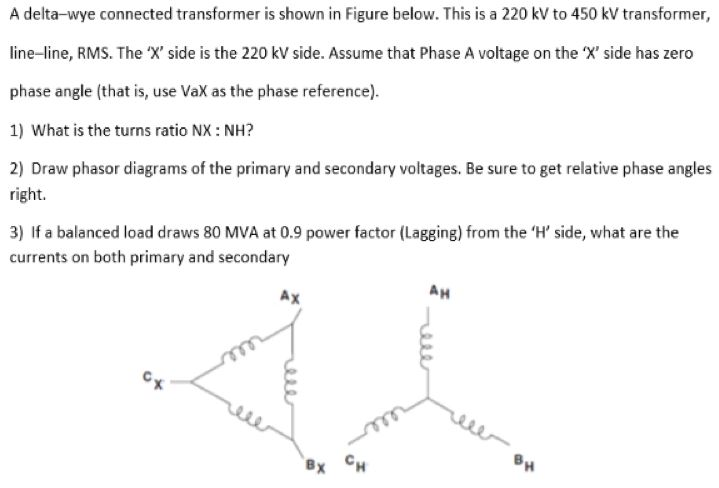A delta-wye connected transformer is shown in Figure below. This is a 220 kV to 450 kV transformer, line-line, RMS. The 'X' side is the 220 kV side. Assume that Phase A voltage on the X' side has zero phase angle (that is, use VaX as the phase reference) 1) What is the turns ratio NX: NH? 2) Draw phasor diagrams of the primary and secondary voltages. Be sure to get relative phase angles right. 3) If a balanced load...

• ### 5. For the Balanced 3-Phase delta () to delta (D) system below: Solve for the line to neutral vol...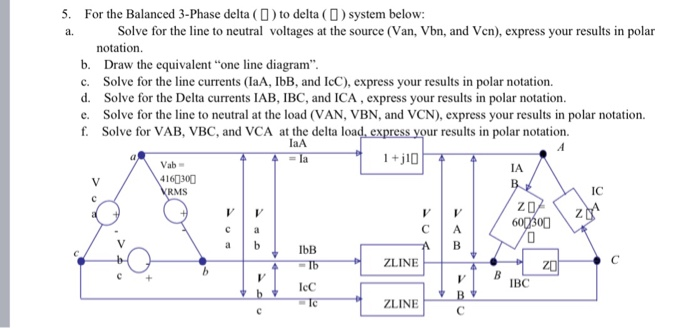5. For the Balanced 3-Phase delta () to delta (D) system below: Solve for the line to neutral voltages at the source (Van, Vbn, and Vcn), express your results in polar notation b. Draw the equivalent "one line diagram c. Solve for the line currents (laA, IbB, and IcC), express your results in polar notation d. Solve for the Delta currents IAB, IBC, and ICA, express your results in polar notation e. Solve for the line to neutral at the...

• ### 09) For the balanced Δ-Y circuit of the Fig. below, calculate the phase and line currents,...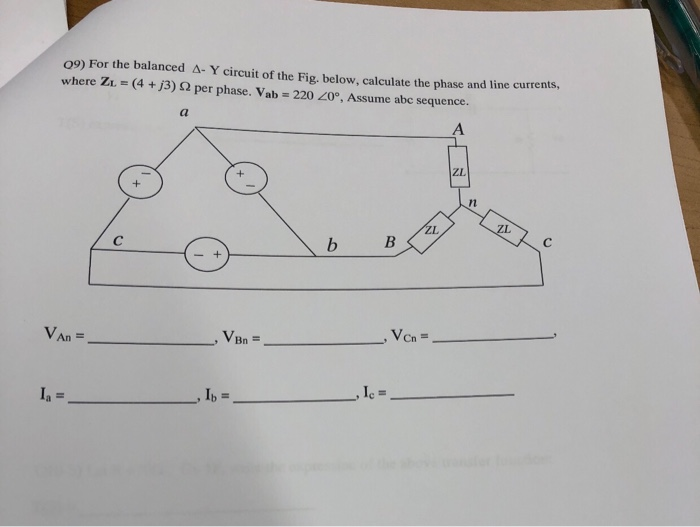09) For the balanced Δ-Y circuit of the Fig. below, calculate the phase and line currents, where Zi- (4 +j3) 2 per phase. Vab 220 20°, Assume abc sequence. ZL Von- Bn

• ### 508 CHAPTER 11 . POLYPHASE CIRCUITS 11.40 In a balanced three-phase system, the source has an...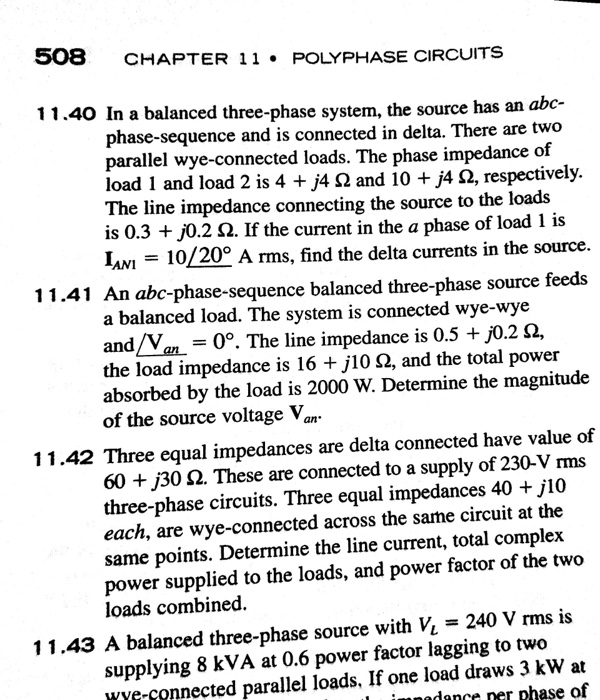508 CHAPTER 11 . POLYPHASE CIRCUITS 11.40 In a balanced three-phase system, the source has an aboc phase-sequence and is connected in delta. There are two rallel wye-connected loads. The phase impedance of load i and load 2 is 4 The line impedance connecting the source to the loads is 0.3 + j0.2 2. If the curre IAN! = 10/20-Arms, find the delta currents in the source 4 Ω and 10 +/4 Ω, respectively. nt in the a phase of...

• ### 2.11. A 4157-V rms, three-phase supply is applied to a balanced Y-connected three-phase load consisting of three id...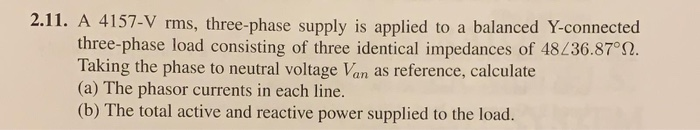2.11. A 4157-V rms, three-phase supply is applied to a balanced Y-connected three-phase load consisting of three identical impedances of 48236.87°2. Taking the phase to neutral voltage Van as reference, calculate (a) The phasor currents in each line. (b) The total active and reactive power supplied to the load.